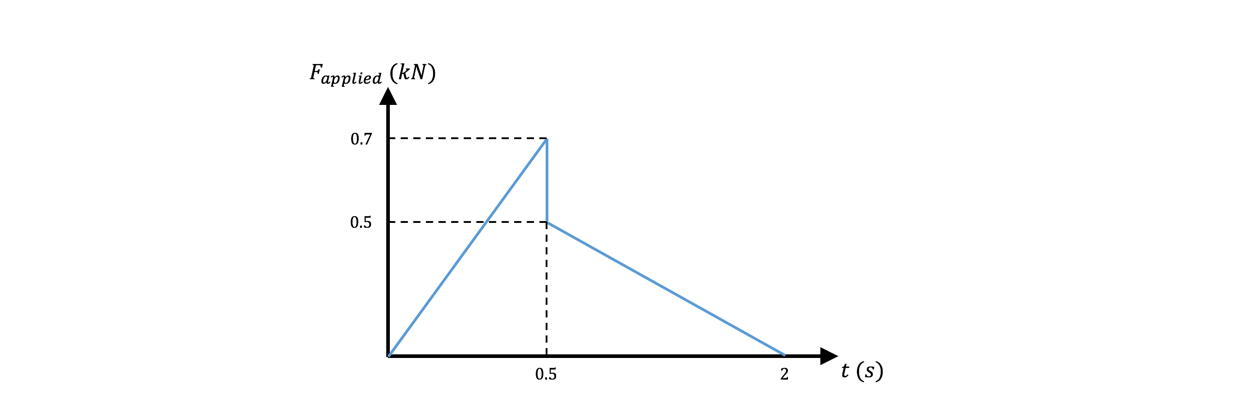# Problem: Consider the figure below, graphing the force applied on a 200 kg box vs time. During the first 0.5 s on the graph, the box remains at rest, while during the remaining time on the graph, the box moves at a constant speed of 15 m/s. Assume that the decrease in the coefficient of kinetic friction is due to changes in the surface the box moves across. How far across this surface does the box have to travel for the coefficient of kinetic friction to reach half its maximum value?

###### Problem Details

Consider the figure below, graphing the force applied on a 200 kg box vs time. During the first 0.5 s on the graph, the box remains at rest, while during the remaining time on the graph, the box moves at a constant speed of 15 m/s. Assume that the decrease in the coefficient of kinetic friction is due to changes in the surface the box moves across. How far across this surface does the box have to travel for the coefficient of kinetic friction to reach half its maximum value?